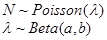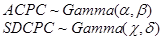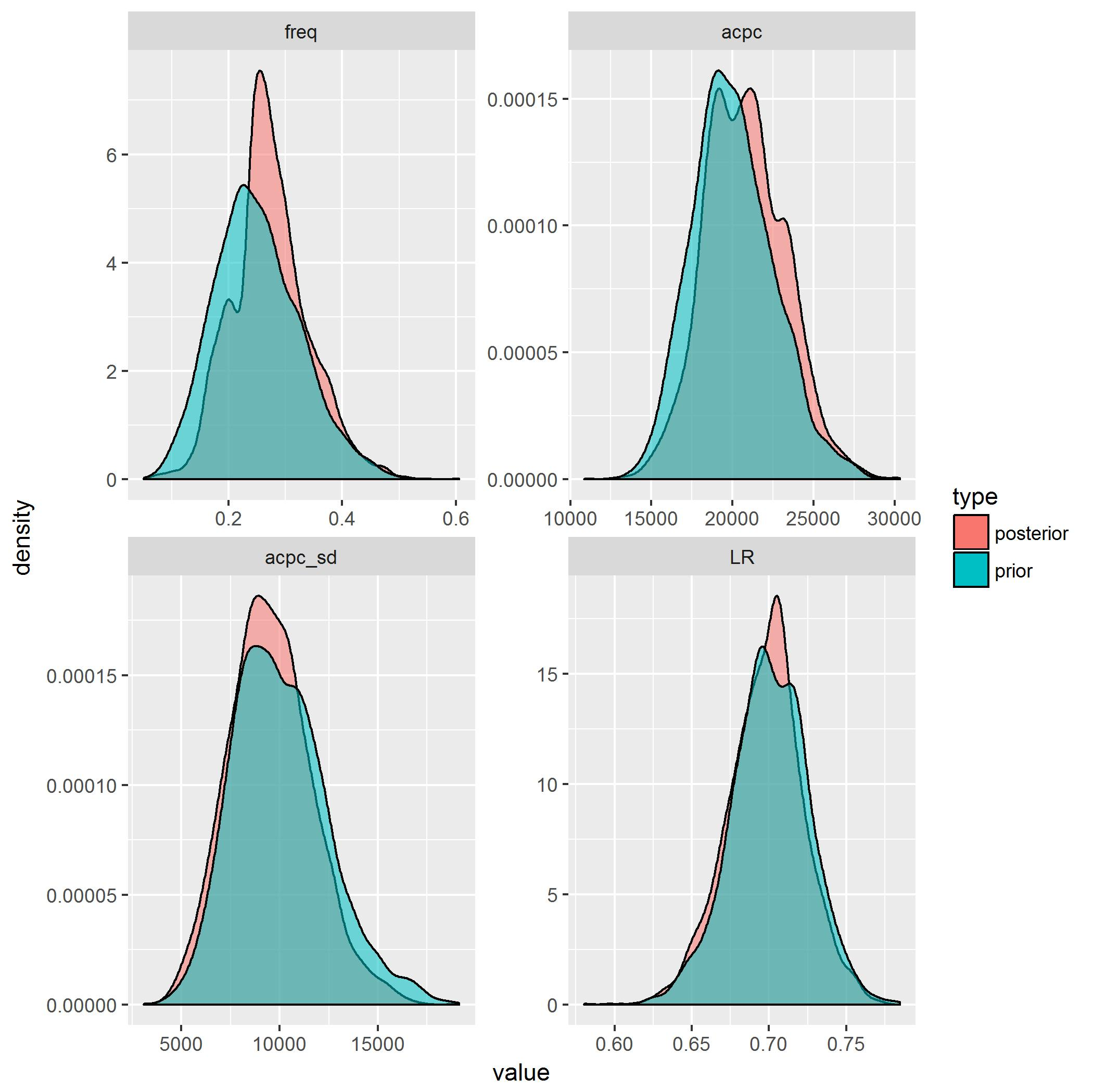Motor policy price comparisons – comparing apples with oranges

Introduction

I recently tried to obtain a quote for comprehensive motor insurance from a price comparison website. The quote was on an older car, worth approximately R70k. After asking for some of my details, the comparison website presented me with something quite similar the following table of premiums and excesses.

Note that these are not the actual premiums and excesses quoted (due to copyright issues) but are modified by adding a normal random variable and then rounding the excesses. I don’t think these changes distort the economic reality of what I was quoted, but, nonetheless, these are not the actual numbers.

 Premium Excess 1 458 9845 2 514 4840 3 534 7620 4 532 4580 5 544 4580 6 584 4580 7 571 4580 8 767 3920 9 894 4515

Most of the policies presented had similar terms and conditions – some sort of cashback benefit, hail cover and car rental. The distinguishing features seemed to be premium and excess. However, as a consumer, in this case, I found it difficult to compare these premiums, except for those with a R4.58k excess. What is a good deal and which of these is more overpriced? It makes some sense that policy number 9 is overpriced – I can get a lower excess for a lower premium, so this policy is definitely sub-optimal. But what about policy 8 – this has a low excess, but seems very expensive compared to the policies with only a slightly higher excess. Is this reasonable? Intuitively, and having some idea how motor policies are priced, my answer is no, but can we show this from the numbers presented?

Moral Soap Box (feel free to skip)

Before getting into the details of how I tried to work with these numbers, I think it is important to stop and consider the public interest. Would the general consumer of insurance have any idea how to compare these different premiums given the different excesses?  Probably not, in my opinion, leading to the title of this post. I guess that some rational consumers would be ‘herded’ into comparing policies 4-7, since they have the same excess, and maybe go for the cheapest one of those. But this is perhaps only a “local minimum” – maybe, in fact, one of the other policies offers better value. Also, one has to rely on the good faith of those running the comparison website to present policies with only the same terms and conditions, or else this supposedly rational strategy might backfire if policy number 4 has worse terms. Lastly, this all makes sense on day one – what will the insurer offering such a generous premium do over the lifetime of the policy – will they keep being so generous or will the consumer be horrified after a couple of steep price hikes.

Hence, this set of quotes seems to me a “comparison of apples with oranges”.

The code

As usual, the code for this post is on my Github, over here:

https://github.com/RonRichman/ABC_pricing/

Note that code is under the open-source MIT License, so please read that if you want to use it!

The theory

Of course, if we had access to the pricing models underlying these premiums then it would be a simple matter to work out what is expensive and what is not, but the companies quoting were not so kind as to share these and only provided these point estimates. I have some ideas about the frequency of occurrence of motor claims and the average cost per claim, so ideally I would want to incorporate this information into whatever calculations I perform, pointing to the need for some sort of Bayesian approach to the problem. However, the issue here is that the price of a general non-Life/P&C policy is really the outcome of a complicated mathematical function – the collective risk process  – often represented by a compound Poisson distribution, which, to my knowledge, does not have an explicit likelihood function (which is why, in practice, actuaries will use Monte Carlo simulation or other approaches like the Panjer approximation or the Fast Fourier Transform to simulate from the distribution). Since most Bayesian techniques require an explicit likelihood function (or the ability to decompose the likelihood function into a bunch of simpler distributions), it would therefore be difficult to build a Bayesian model with standard methods like Markov Chain Monte Carlo (MCMC).

So, in this blog post I share an approach to this problem that I took using an amazing technique called Approximate Bayesian Computation (‘ABC’). To explain the basic idea, it is worth going back to the basics of Bayesian calculations, which try to make direct inferences about parameters in a statistical problem. These calculations generally progress in three steps

• Prior information on the problem at hand is encoded in a statistical distribution for the parameters we are interested in. For example, the average cost per claim might be distributed as a Gamma distribution.
• The data likelihood is then calculated based on a realization of the parameters from the prior distribution.
• The likelihood of a set of parameters is then assessed as the product of a) the likelihood of getting that parameter set multiplied with b) the data likelihood divided by c) the total probability of all parameter sets and data likelihoods.

In this case, the data likelihood is not available easily. The basic idea of ABC is that in models with an intractable likelihood function, one can use a different method of ascertaining whether or not a parameter set is “likely” or not. That is, by generating data based on the prior distribution and comparing how “close” this generated data is to the actual data, one can get a feel for which parts of the prior distribution make sense in the context of the data, and which do not.

For some more information on ABC, have a look at this blog post and the sources it quotes:

http://www.sumsar.net/blog/2014/10/tiny-data-and-the-socks-of-karl-broman/

The generative model and priors

I assumed that the number of claims, N, claims are distributed as a Poisson distribution, with a frequency parameter drawn from a beta distribution:I selected the parameters of the Beta distribution to produce a mean frequency of .25 (i.e. a claim every four years) with a standard deviation of .075.

Cost per claim was modelled as a log-normal distribution:Instead of putting priors on   and , which do not have an easy real world interpretation, instead I chose priors for the average cost per claim (ACPC) and the standard deviation of the cost per claim (SDCPC) , and, for each draw from these prior distributions, found the matching parameters for the log-normal. Both of these priors were modelled as Gamma distributions:with the parameters of the gamma chosen so that the average cost per claim is R20k with a standard deviation of R2.5k and the standard deviation of the cost per claim is R10k with a standard deviation of R2.5k.

The code to find the corresponding log-normal parameters, once we have an ACPC and SDCPC is:

lnorm_par = function(mean, sd) {

cv = sd/mean

sigma2 = log(cv^2+1)

mu = log(mean)-sigma2/2

results = list(mu,sigma2)

results

}

Lastly, I assumed that the insurers are working to a target loss ratio of 70% (i.e. for every 70c of claims paid, the insurers will bring in R1 of income), with a standard deviation of 2.5%. This distribution also followed a beta, similar to the frequency rate.

The following algorithm was then run 100 000 times:

• Draw a frequency parameter from the Beta prior
• Simulate the number of claims from the Poisson distribution, using the frequency parameter
• Draw an average cost per claim and it’s standard deviation, and find the corresponding log-normal distribution
• For each claim, simulate a claim severity from the log-normal
• For each excess with a corresponding premium quote, subtract the excess from the claims and add these up
• The implied premium is the sum of the claims net of the excess divided by:
• 12, since we are interested in comparing monthly premiums
• the target loss ratio of the insurers, to gross up the premium for expenses and profit margins

Inference

So far we have generated lots of data from our priors. Now it is time to see which of the parameter combinations actually produce premiums reasonably in line with the quotes on the website. To simplify things, I put each of the simulated parameters into one of nine “buckets” depending on the percentile of the parameter within its prior distribution.

claims[,freq_bin :=ntile(freq,9)]

claims[,sev_bin :=ntile(acpc,9)]

claims[,sev_sd_bin :=ntile(acpc_sd,9)]

claims[,lr_bin :=ntile(LR,9)]

claims[,id:=paste0(freq_bin, sev_bin, sev_sd_bin, lr_bin)]

Then, indicative premiums for each bucket were derived by averaging the premiums derived in the previous section for each parameter “bucket”. The distance between the generated data and the actual quoted premiums was taken as the absolute percentage error:

And for the very last step, the median distance between the generated and quoted premiums was found for each parameter bucket. I only selected those “buckets” which produced a median distance of less than 8%. The median was used, instead of the mean, since I believe that some of the quotes are actually unreasonable, and I do not want to move the posterior distance too much in their favour by using a distance metric that is sensitive to outliers.

Now we have everything we need to show the posterior distributions of the parameters:Some observations are that the prices I was quoted implies both a frequency and severity of claims that are a little bit higher than I assumed, but with a lower average cost per claim. The standard deviation of the average cost per claim is lower as well, with less weight given to the tails than I had assumed. Lastly, the loss ratio distribution matches the prior quite well.

Prices

Lastly, the implied prices are shown in red the next image.Bearing in mind that this is all based on the assumption of actuarially unfair premiums – in other words, allowing the insurer to add a substantial profit to the actual risk premium by targeting a loss ratio of 70% – only three of the quotes are reasonable (two of those with an excess of R4.58k and the one with an excess of R4.84k). The rest of the quotes are significantly higher than can be justified by my priors on the key elements of the claims process, and it would seem irrational for a consumer with similar priors to take out one of these policies.

Conclusion

This post showed how it is possible to back out the parameters that underlie an insurance quote using prior information and Approximate Bayesian Computation.  Based on the analysis, we can go back to the original question I asked at the beginning of the post – is the low excess policy number 8 priced reasonably? The answer, based on my priors, seems to be “no”, and the excesses quoted here do not seem to be all that useful when it comes to explaining the prices of each quote.

What could be modelled more accurately? Some of the policies include a cashback, which we could priced explicitly using the posterior parameter distributions, but I personally attach very little utility to cashback benefits and would not pay more for one. So this is a more minor limitation, in my opinion.

I would love to hear your thoughts on this.